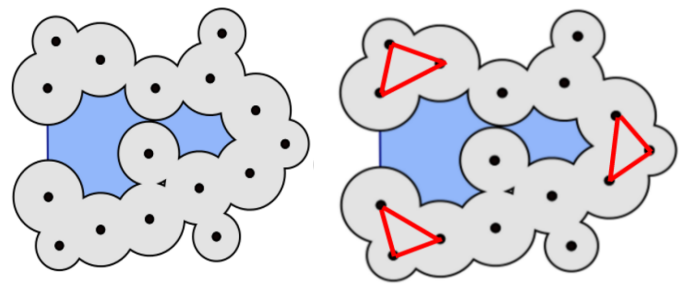#### Efficient parallel algorithm to compute a sub-complex of the weighted Delaunay triangulation for molecular data.

Ranjanikar Nikhil Prabhakarrao

#### Abstract

In the field of bio-molecules, it is utmost important to study the behaviour of a molecule while interacting with another. This interaction decides the functionality of the molecule. It is widely accepted fact that the geometrical shape of bio-molecules determines their functionality. Using alpha complex and regular triangulation, one can easily calculate most of the geometric parameters of a molecule. Volume of a molecule and many other things can be computed using alpha complex. In most of the applications, alpha complex corresponding to alpha values 0 and 1.4, is required. Here, the application requires a small sub-set of the complete triangulation. However, to get that small sub-set, we have to bear additional over head of computation of the whole triangulation. Hence, we propose a novel approach which can directly compute the sub-complex or sub-set of the triangulation without generating the whole triangulation.

We propose an algorithm to compute the sub-complex of a regular triangulation(RT) for 3D molecular data using localized properties. In this paper, we have implemented an incre- mental algorithmic approach, which builds a smaller tetrahedra first and then a larger one, to compute sub-complex of RT that is parametrized by a real value α. We have used edge and alpha optimization to reduce time complexity of algorithm. Our algorithm can exploit massive parallelism supported by GPUs in order to construct RT. For getting maximum advantage at an architectural level, we used three optimizations. Pinned memory and CUDA streams are used to reduce the data transfer time while texture memory is used to reduce memory access time.

All of these optimizations reduce the execution time of our algorithm by 27%. We obtain upto 88% improvement in execution time for smaller alpha value, in computing a sub-complex, over an existing state-of-art method gReg3d, which computes complete triangulation. We obtain speedup upto 9x over best sequential CPU implementation, CGAL for zero alpha value.

[PDF]